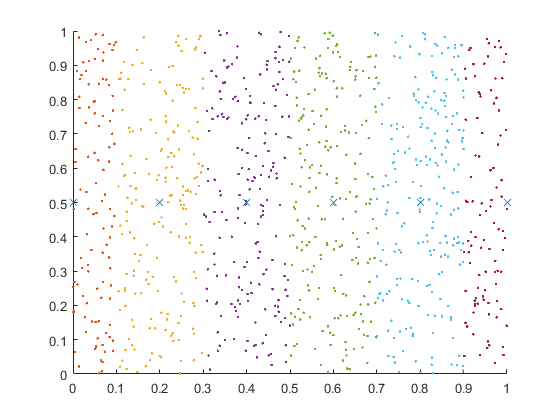Uniqueness and Membership

We have had many requests over the years for some of our functions to include tolerances, e.g., find. I don't know if you noticed, but some of the relevant functions (not find) now allow this.

Contents

uniquetol and ismembertol

Recently (R2015a), we added ismembertol and uniquetol to MATLAB. This is to reflect the notion that, especially with floating point arithmetic, we should not generally expect quantities that we compare to be exact matches, but close, to within an tolerance. You can argue that |find|ing something might sound a bit more specific, hence no tolerance there. Not sure if that holds up. And, of course, there's always eps. So now there are some more good tools for you to use!

Specify Data Scaling by Column

Let's suppose we have some data that we want to group into different vertical bands. The rows of A and B contain the x and y values of the points. Because of this, we set 'ByRows' to be true. And we want all points included; hence 'OutputAllIndices' is also true. Finally we use the 'DataScale' to be unbounded at the high end for y so we can ignore those values, but 1 for an absolute tolerance for x.

A = rand(1000,2);
B = [(0:.2:1)',0.5*ones(6,1)];
[LIA,LocAllB] = ismembertol(B, A, 0.1, 'ByRows', true, ...
'OutputAllIndices', true, 'DataScale', [1,Inf])
LIA =
1
1
1
1
1
1
LocAllB =
[103x1 double]
[185x1 double]
[188x1 double]
[213x1 double]
[212x1 double]
[ 99x1 double]

You can see we get several bands of data, with different amounts in each bin of LocallB.

Plot the Results

Now we want to plot the points in A that are within the distance of the stated tolerance for each point in B.

hold on
plot(B(:,1),B(:,2),'x')
for k = 1:length(LocAllB)
plot(A(LocAllB{k},1), A(LocAllB{k},2),'.')
end
hold off